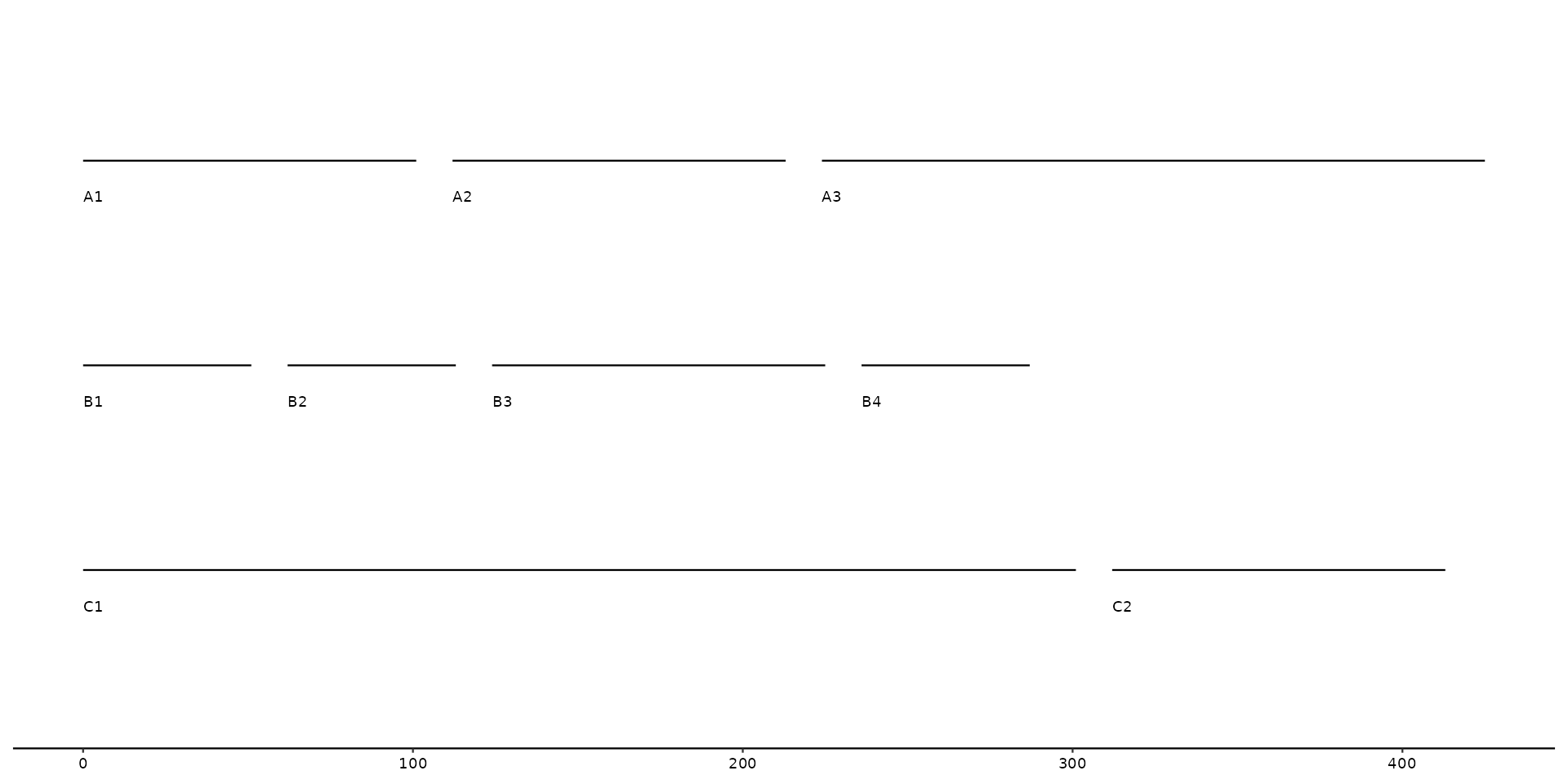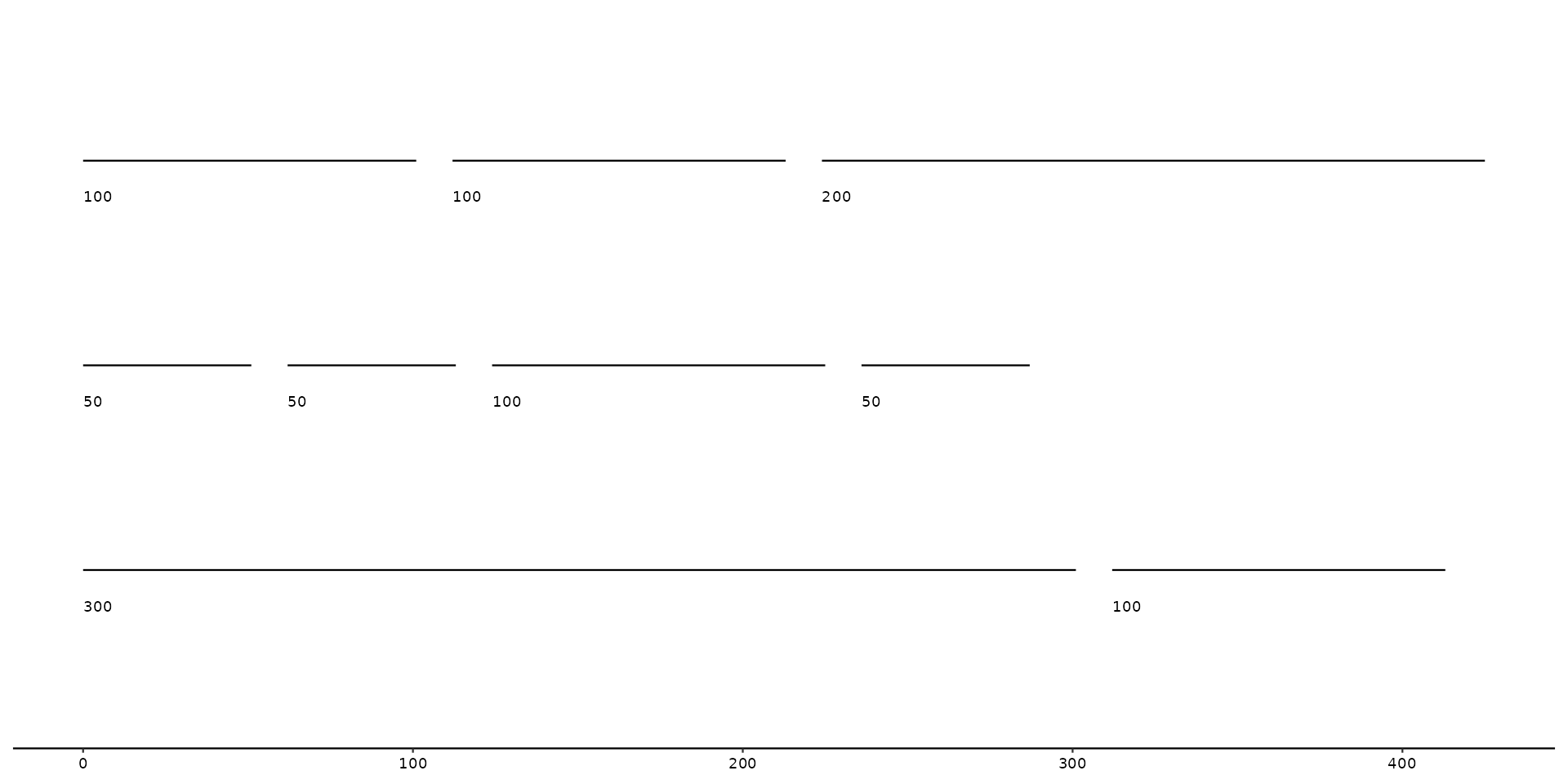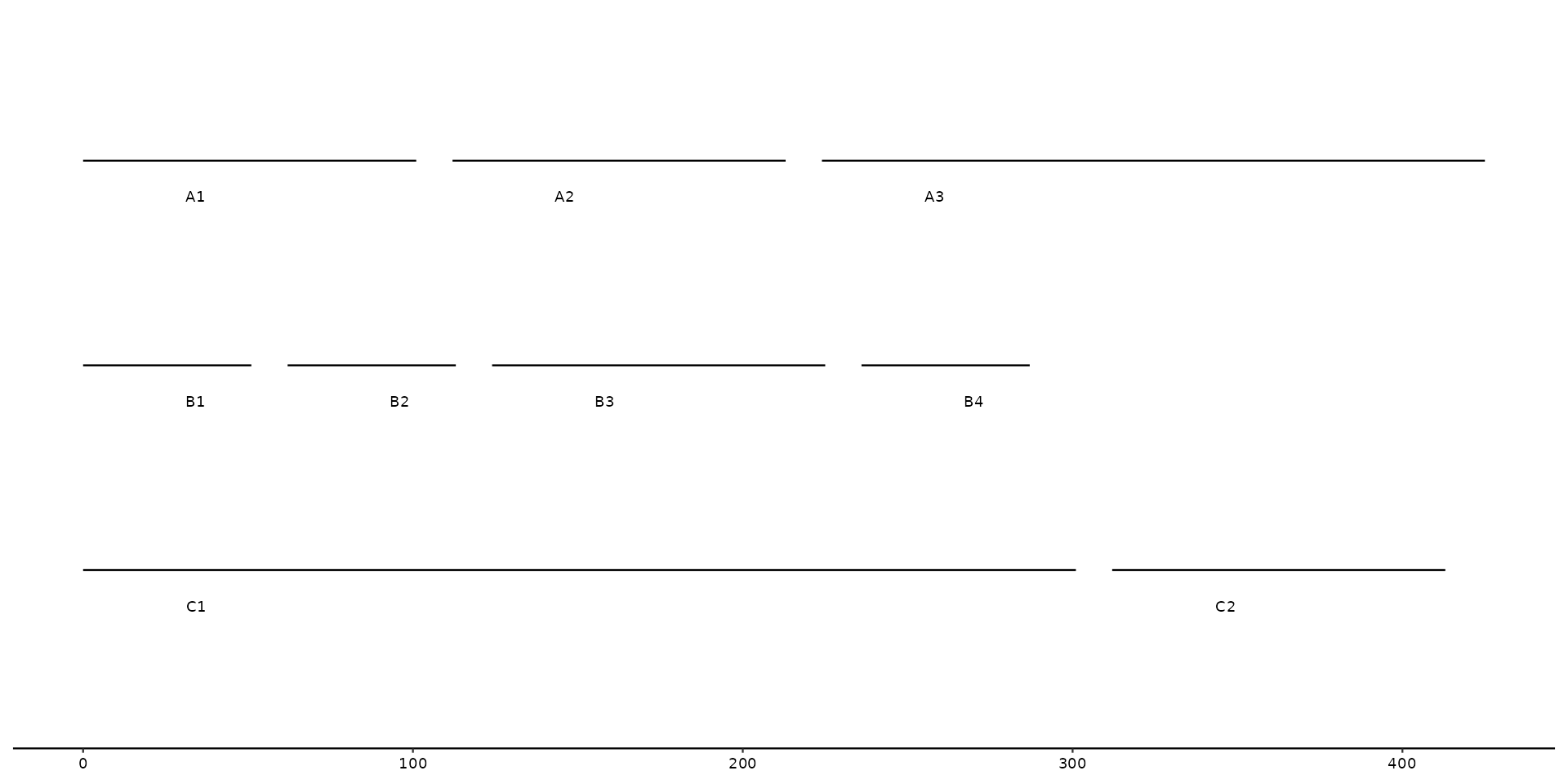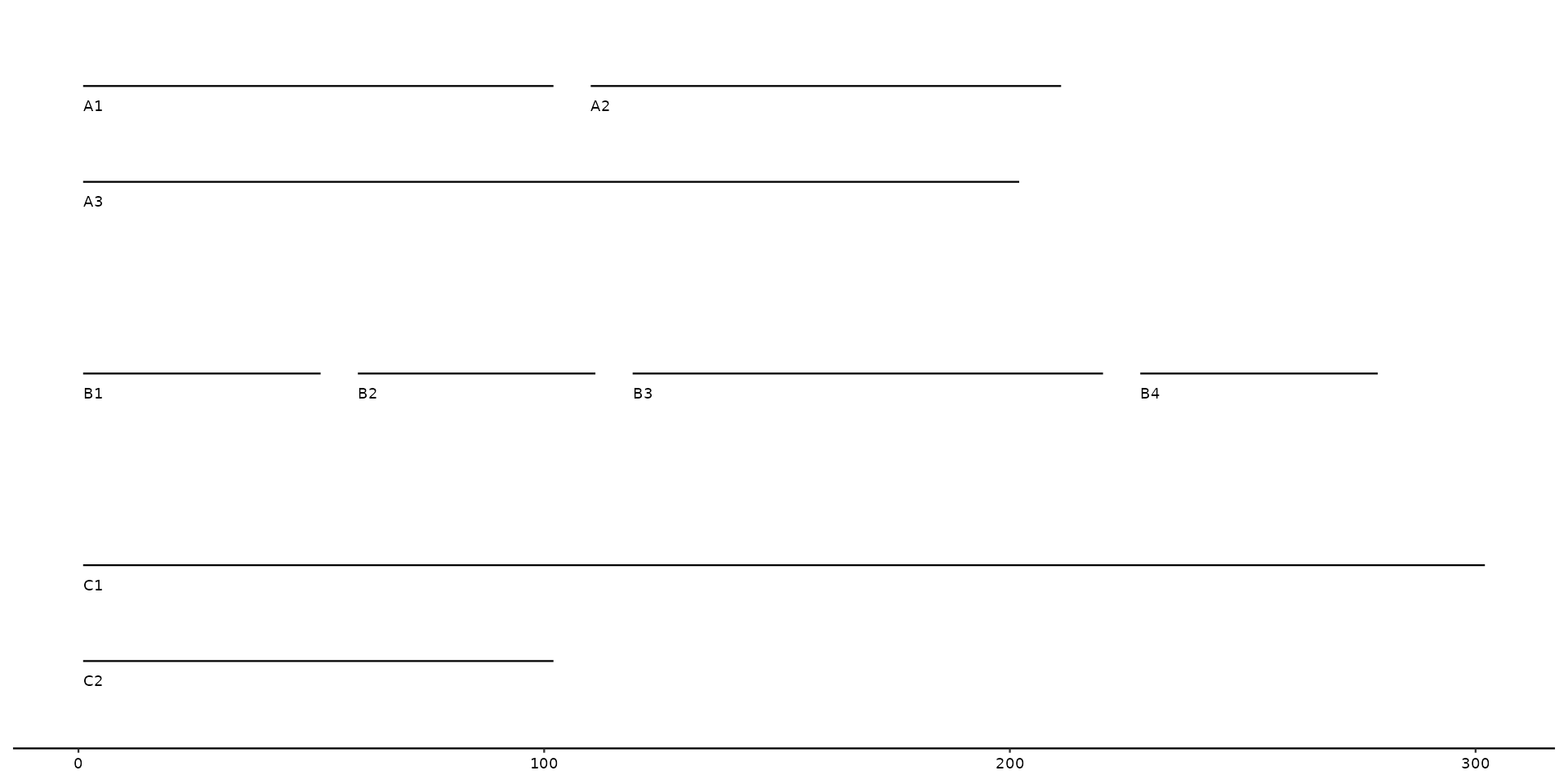This function will put labels at each individual sequence. By default it will plot the seq_id as label, but users are able to change this manually.

Position of the label/text can be adjusted with the different arguments (e.g. vjust, hjust, angle, etc.)

geom_seq_label(
mapping = NULL,
data = seqs(),
hjust = 0,
vjust = 1,
nudge_y = -0.15,
size = 2.5,
...
)

## Arguments

mapping

Set of aesthetic mappings created by aes(). If specified and inherit.aes = TRUE (the default), it is combined with the default mapping at the top level of the plot. You must supply mapping if there is no plot mapping.

data

The data to be displayed in this layer. There are three options:

If NULL, the default, the data is inherited from the plot data as specified in the call to ggplot().

A data.frame, or other object, will override the plot data. All objects will be fortified to produce a data frame. See fortify() for which variables will be created.

A function will be called with a single argument, the plot data. The return value must be a data.frame, and will be used as the layer data. A function can be created from a formula (e.g. ~ head(.x, 10)).

hjust

Moves the text horizontally

vjust

Moves the text vertically

nudge_y

Moves the text vertically an entire contig/sequence. (e.g. nudge_y = 1 places the text to the contig above)

...

Other arguments passed on to layer(). These are often aesthetics, used to set an aesthetic to a fixed value, like colour = "red" or size = 3. They may also be parameters to the paired geom/stat.

## Details

This labeling function uses ggplot2::geom_text() under the hood. Any changes to the aesthetics of the text can be performed in a ggplot2 manner.

## Examples

# example data
seqs <- tibble::tibble(
bin_id = c("A", "A", "A", "B", "B", "B", "B", "C", "C"),
seq_id = c("A1", "A2", "A3", "B1", "B2", "B3", "B4", "C1", "C2"),
start = c(0, 100, 200, 0, 50, 150, 250, 0, 400),
end = c(100, 200, 400, 50, 100, 250, 300, 300, 500),
length = c(100, 100, 200, 50, 50, 100, 50, 300, 100))

# example plot using geom_seq_label
gggenomes(seqs = seqs) +
geom_seq() +
geom_seq_label()# changing default label to length column
gggenomes(seqs = seqs) +
geom_seq() +
geom_seq_label(aes(label=length))